Try out our new practice tests completely free!# Quiz 7: BJT Power Amplifiers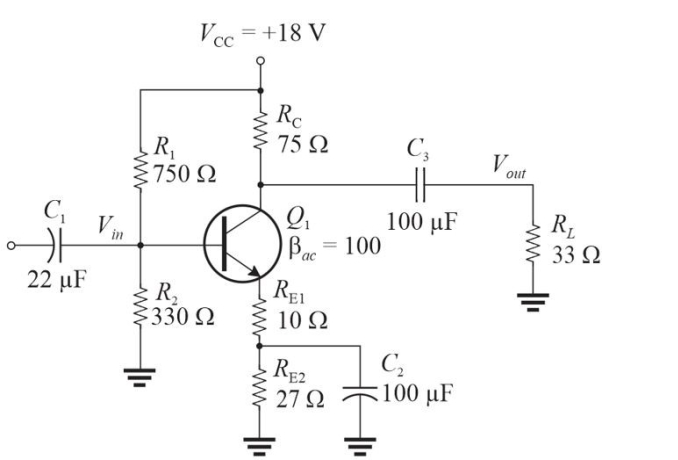Figure 1 -Refer to Figure 1. The voltage gain, Av, to the load is approximately(Multiple Choice)

DThe amplifier type that frequently has a resonant circuit load is(Multiple Choice)

BThe amplifier type that has the worst efficiency is(Multiple Choice)

BIn a single- stage class- A amplifier, the quiescent current is the same as the
(Multiple Choice)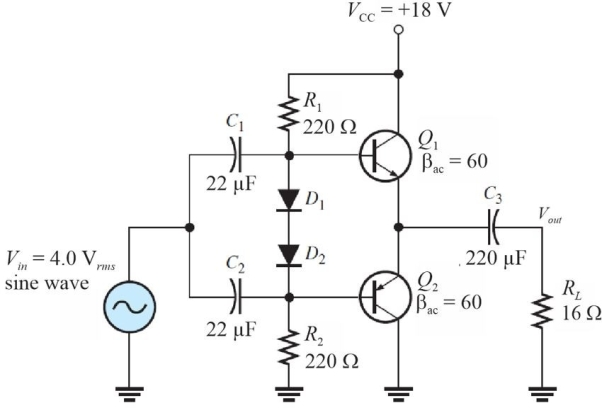Figure 7 -Refer to Figure 7. The expected dc emitter voltage for Q1 is
(Multiple Choice)A heat sink is used to
(Multiple Choice)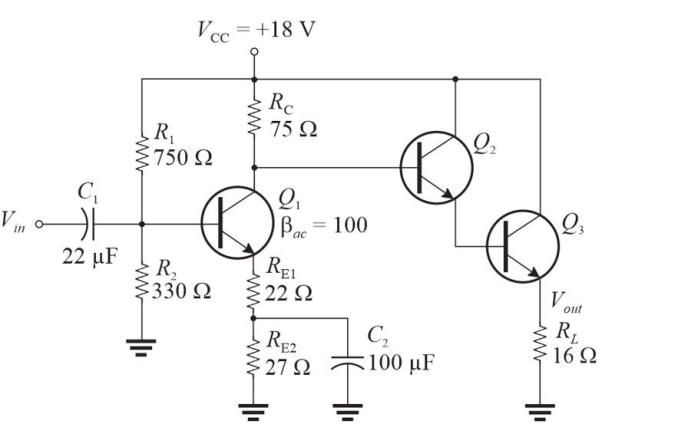Figure 2 This is modification of Figure 1 (added Q2, Q3, and RL, deletion of C3 and a changed value of RE1). -Refer to Figure 2. With no applied signal, the dc power dissipated in the load is approximately
(Multiple Choice)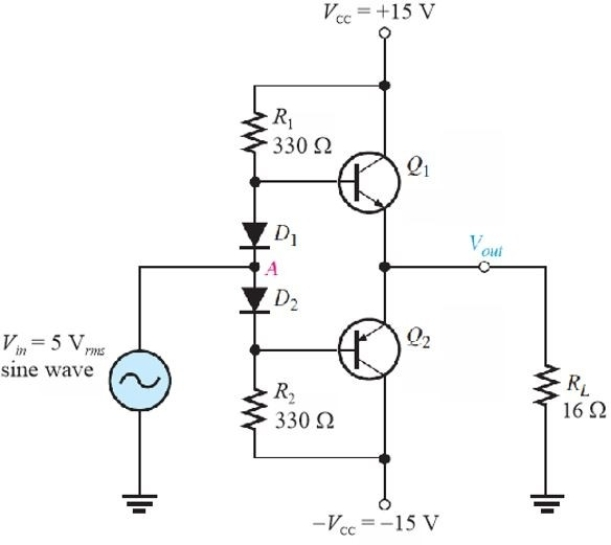Figure 3 -Refer to Figure 3. Note the input is a 5 Vrms sine wave. The ac power in RL is ideally
(Multiple Choice)A class- A amplifier has an input resistance of 520 ▲ and a load resistance of 26 ▲. Assume a 1 Vrms input produces a 5 Vrms output The power gain is
(Multiple Choice)Figure 2 This is modification of Figure 1 (added Q2, Q3, and RL, deletion of C3 and a changed value of RE1). -Refer to Figure 2. If Vin = 1 Vrms, the ac power in RL is approximately
(Multiple Choice)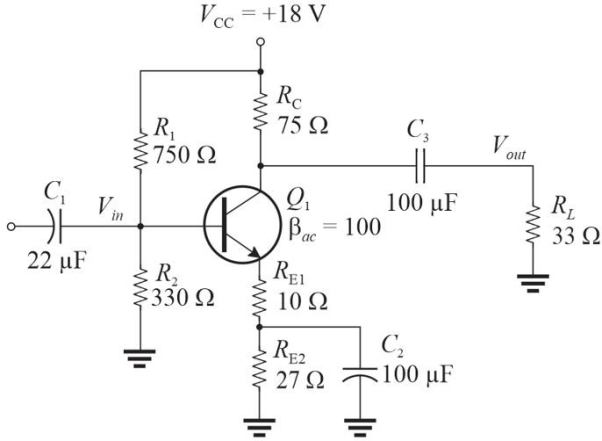Figure 1 -Refer to Figure 1. The input impedance is approximately
(Multiple Choice)Figure 3 -Refer to Figure 3. The value of dc bias current in R1 is
(Multiple Choice)Figure 3 -Refer to Figure 3. With no signal in, IC(Q1)is ideally
(Multiple Choice)Assume the Q point on a class- A amplifier is centered on the ac load line. The maximum peak- to- peak output voltage is
(Multiple Choice)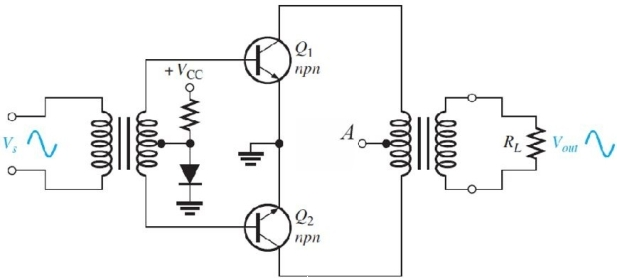Figure 5 -Refer to Figure 5. The diode
(Multiple Choice)Figure 7 -Refer to Figure 7. The power delivered to the load is
(Multiple Choice)Figure 1 -Refer to Figure 1. The power gain, Ap, to the load is approximately
(Multiple Choice)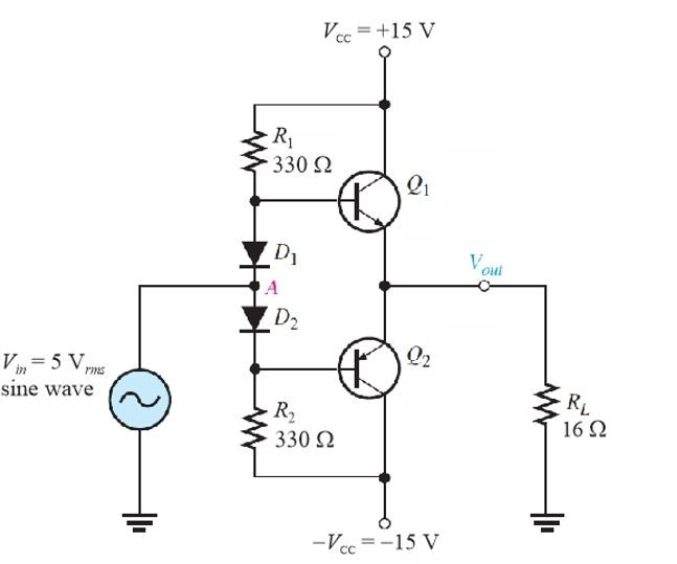Figure 3 -Refer to Figure 3. This type of bias is called
(Multiple Choice)Figure 5 -Refer to Figure 5. The center tap on the output transformer (markedA)should be connected to
(Multiple Choice)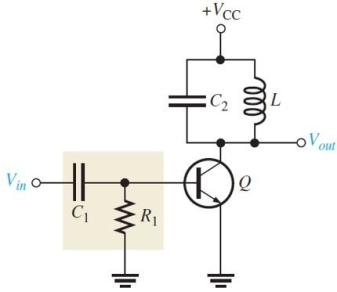Figure 6 -Refer to Figure 6. The combination of R1, C1, and the base- emitter diode form a
(Multiple Choice)## Filters

• Essay(0)
• Multiple Choice(0)